# 6th class maths Decimals exercise 8.6 solutions Ncert

Maths Ncert Class 6 textbook Chapter 8 Decimals Exercise 8.6 solutions are given.

Study the textbook lesson Decimals very well.

Observe the example problems and solutions given in the textbook.

Observe the given solutions and try them in your own method.

You can see

M

# Chapter 8 Exercise 8.6 solutions class 6 Ncert Maths

Chapter – 8

Decimals

Exercise 8.6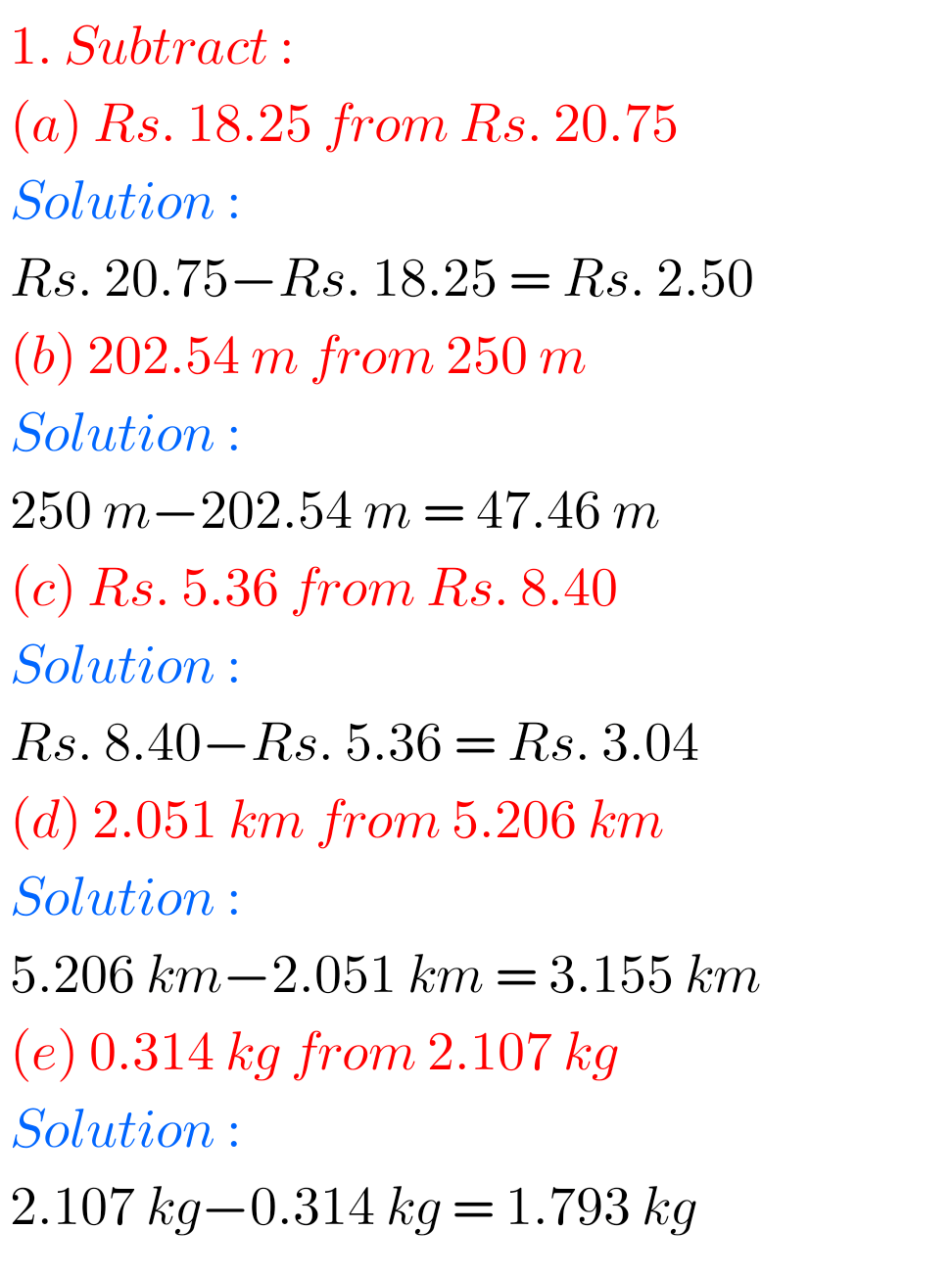## Solutions for Decimals Class 6 Ncert Maths exercise 8.6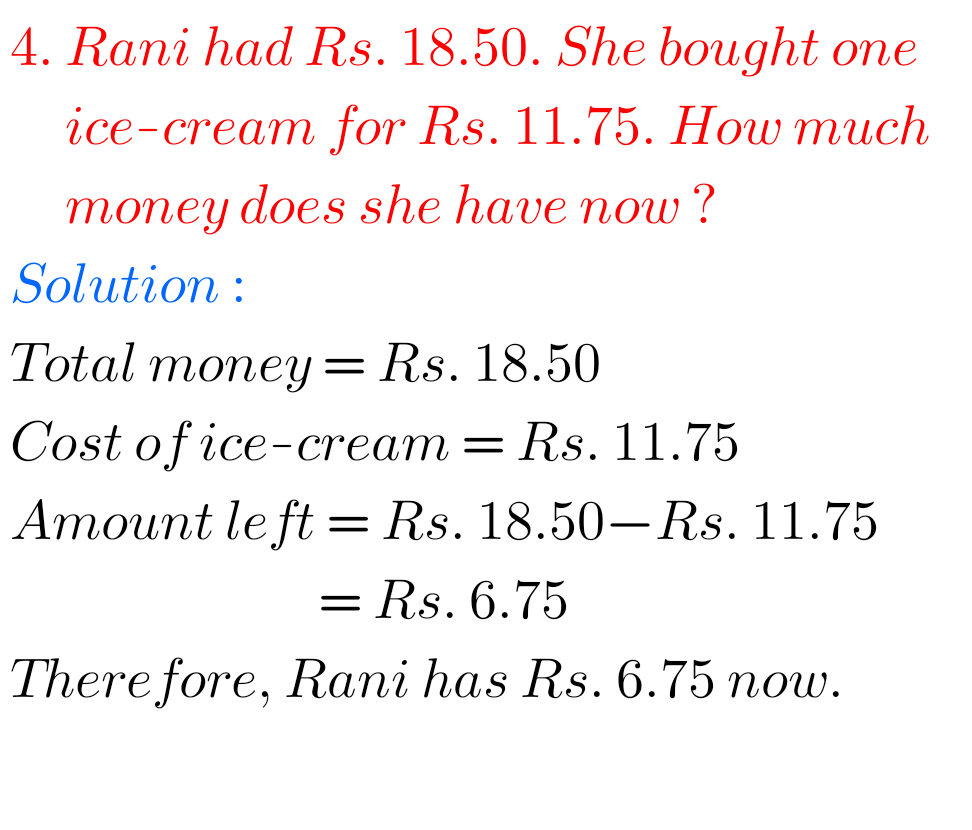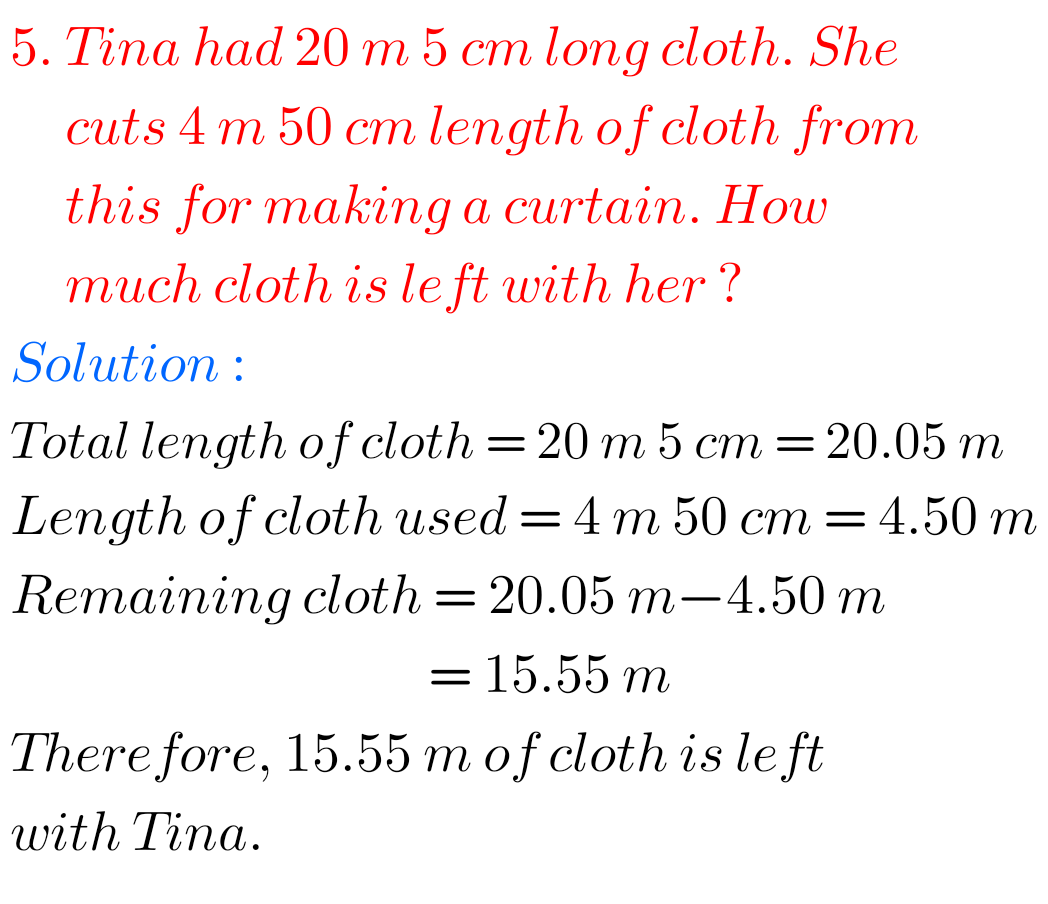## Class 6 maths Ncert Exercise 8.6 solutions Decimal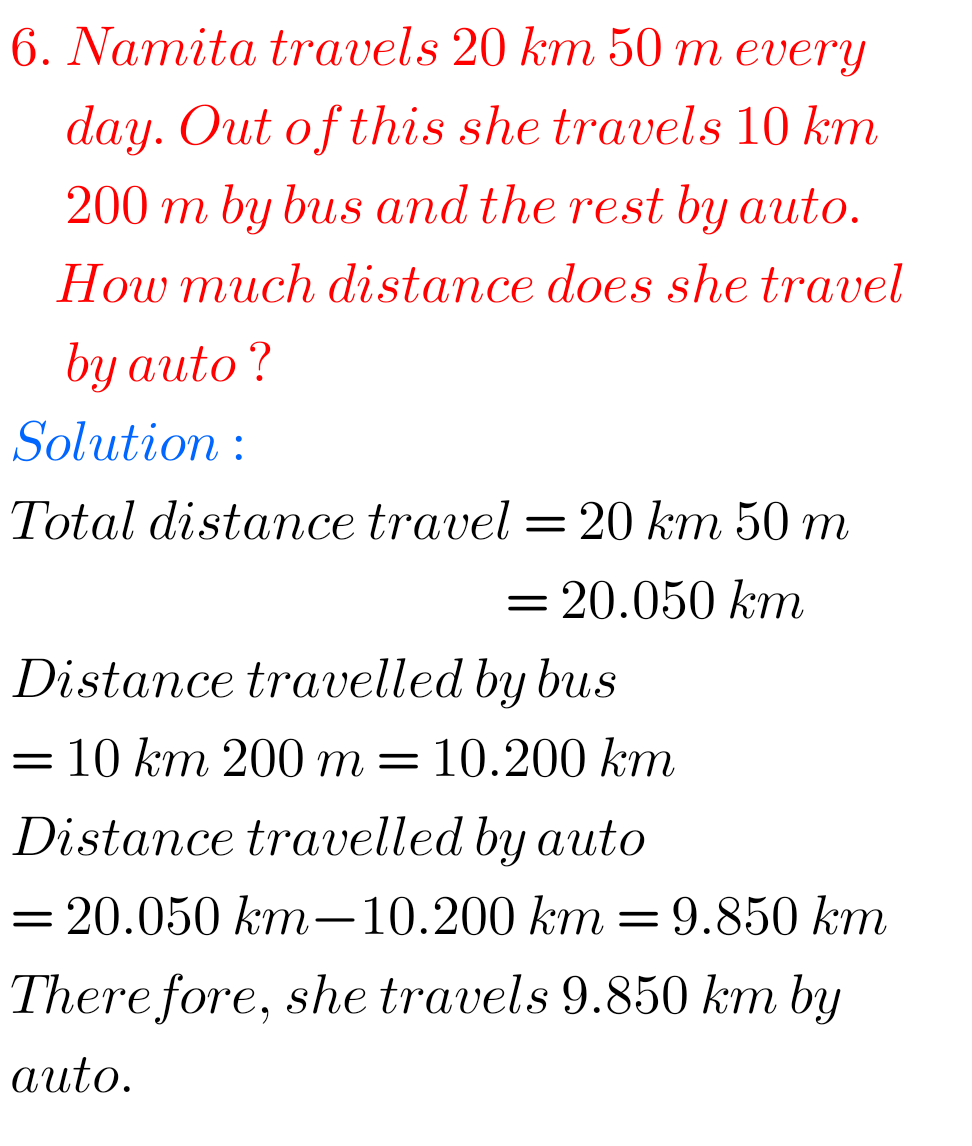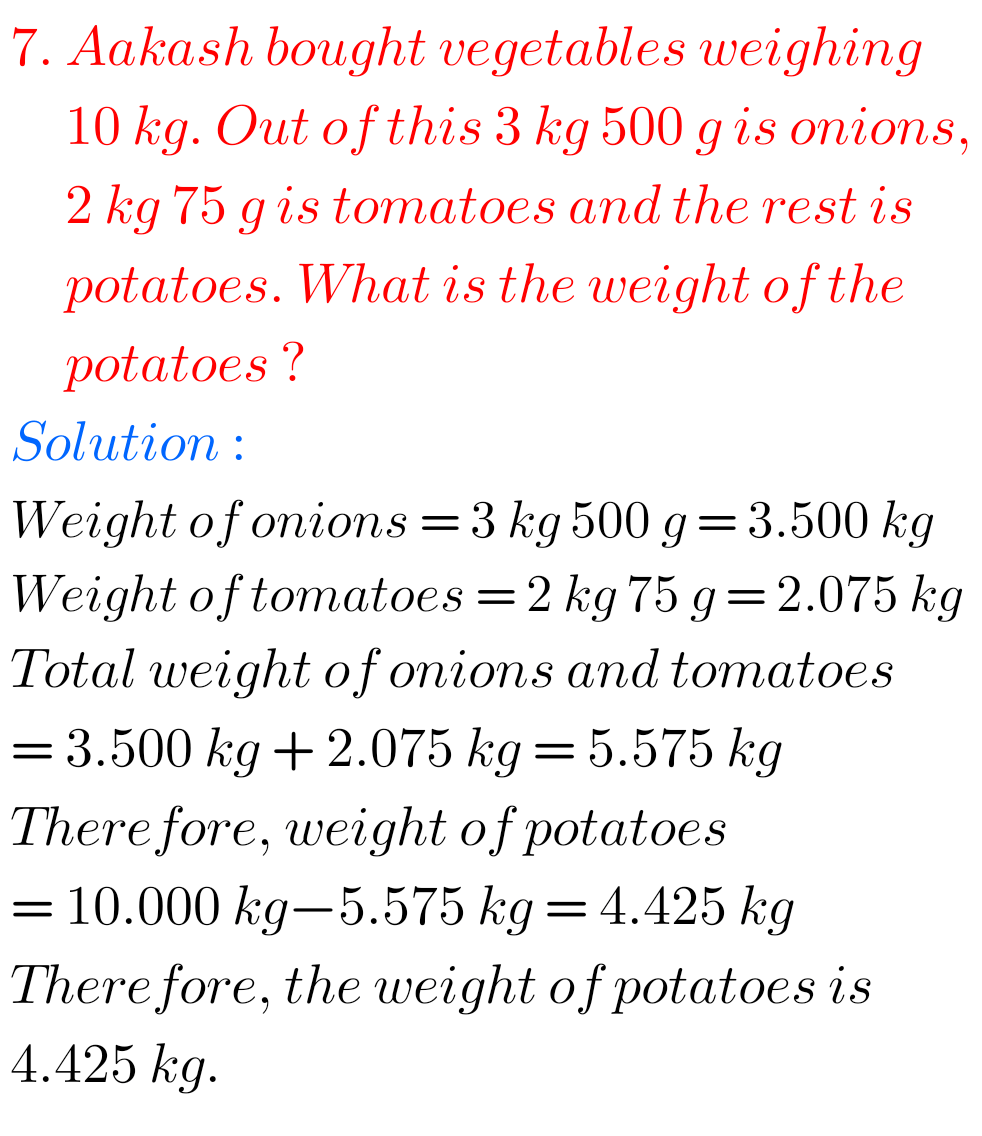Note : Observe the solutions and try them in your own method.